# Three-parameter model transformations of the Platonic Solids faces

Three parameters are used to transform Platonic Solids faces: t - for truncating, q-similarity transformations, α-angle rotation of faces. With these parameters, using the well-known Expansion and Snub operations applied to a polyhedron, you can explore various polyhedra and get a number of Archimedean Solids. Table of the Archimedean Solids obtained in three-parameter models of transformations of the Platonic Solids faces: Platonic Solids models Tetrahedron Octahedron Cube Icosahedron Dodecahedron Archimedean Solids 1: Truncated Tetrahedron + 2: Cuboctahedron + + + 3: Truncated Octahedron + + + 4: Truncated Cube + + 5: Rhombicuboctahedron + + 6: Snub Cube + + 7: Icosidodecahedron + + 8: Truncated Cuboctahedron + + 9: Truncated Icosahedron + + 10: Truncated Dodecahedron + + 11: Rhombicosidodecahedron + + 12: Snub Dodecahedron + + 13: Truncated Icosidodecahedron + +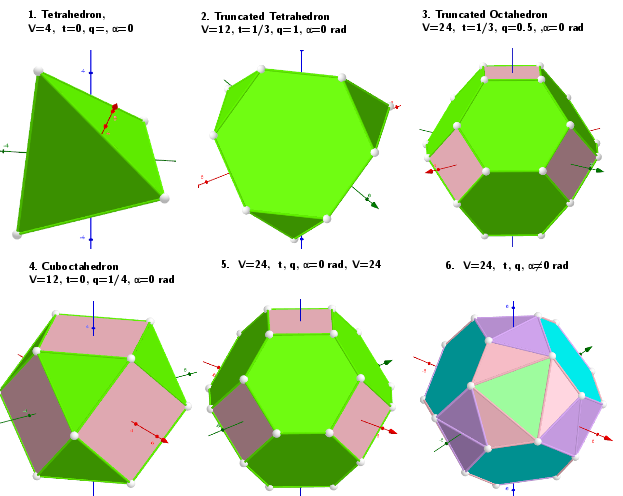The values of the parameters in the Tetrahedron-model that defines a well-known polyhedra.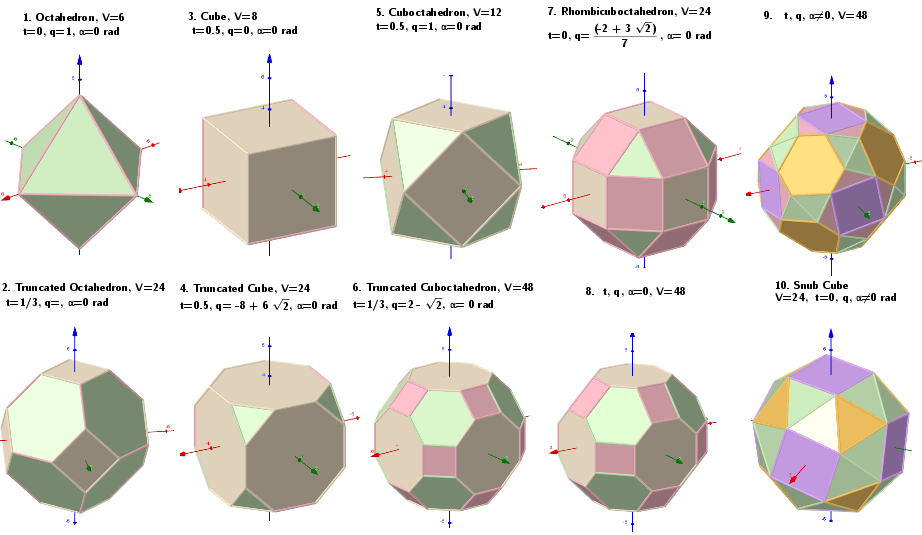The values of the parameters in the Octahedron-model that defines a well-known polyhedra.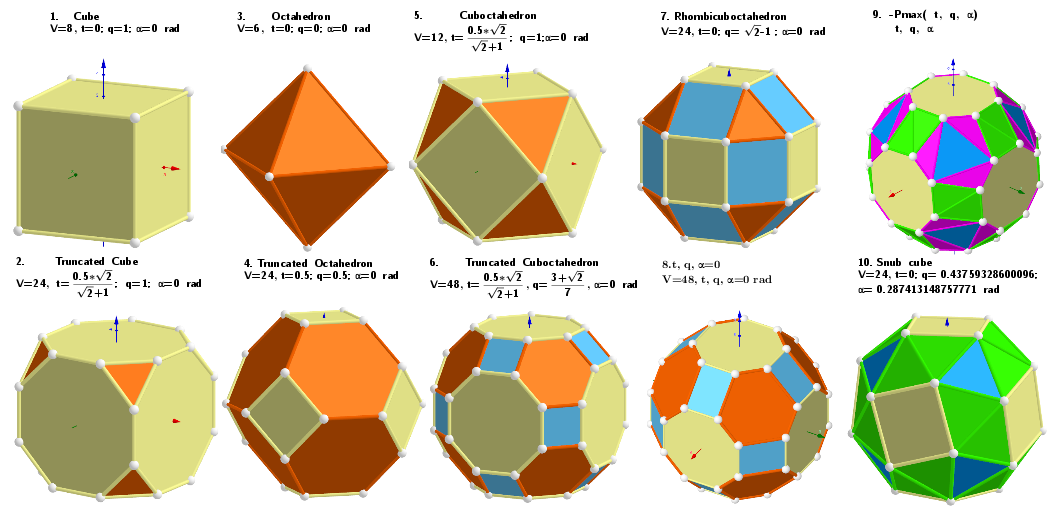The values of the parameters in the Cube-model that defines a well-known polyhedra.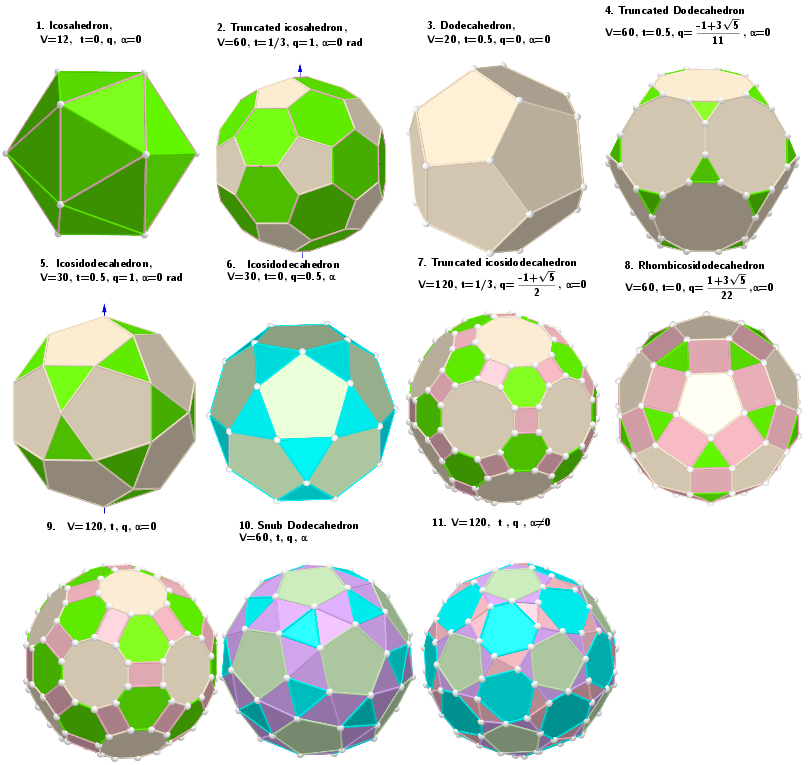The values of the parameters in the Icosahedron-model that defines a well-known polyhedra.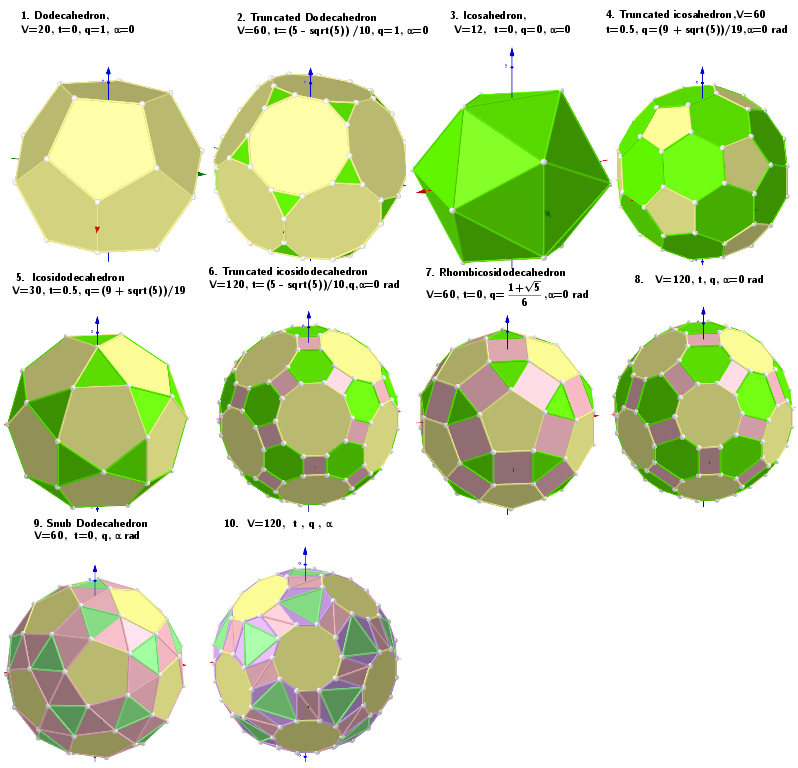The values of the parameters in the Dodecahedron-model that defines a well-known polyhedra.